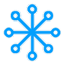#创客百科

### 侧边栏

• 树莓派瑞士军刀扩展板

Arduino

MicroPython

micro:bit

en:arduino:language:int

# int

## Description

Integers are your primary datatype for number storage, and store a 2 byte value. This yields a range of -32,768 to 32,767 (minimum value of -2^15 and a maximum value of (2^15) - 1).

Int's store negative numbers with a technique called 2's complement math. The highest bit, sometimes refered to as the “sign” bit, flags the number as a negative number. The rest of the bits are inverted and 1 is added.

The Arduino takes care of dealing with negative numbers for you, so that arithmetic operations work transparently in the expected manner. There can be an unexpected complication in dealing with the bitshift right operator (») however.

## Example

`    int ledPin = 13;`

## Syntax

`    int var = val;`
• var - your int variable name
• val - the value you assign to that variable

## Coding Tip

When variables are made to exceed their maximum capacity they “roll over” back to their minimum capacitiy, note that this happens in both directions.

```   int x
x = -32,768;
x = x - 1;       // x now contains 32,767 - rolls over in neg. direction

x = 32,767;
x = x + 1;       // x now contains -32,768 - rolls over```

en/arduino/language/int.txt · 最后更改: 2016/12/25 22:15 (外部编辑)

### 页面工具